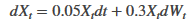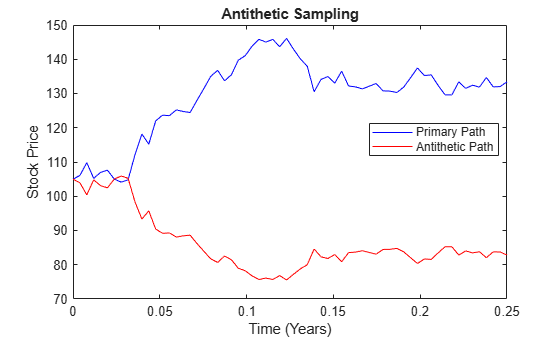# simulate

Simulate multivariate stochastic differential equations (SDEs) for `SDE`, `BM`, `GBM`, `CEV`, `CIR`, `HWV`, `Heston`, `SDEDDO`, `SDELD`, `SDEMRD`, `Merton`, or `Bates` models

## Syntax

``[Paths,Times,Z] = simulate(MDL)``
``[Paths,Times,Z] = simulate(___,Optional)``

## Description

example

````[Paths,Times,Z] = simulate(MDL)` simulates `NTrials` sample paths of `NVars` correlated state variables, driven by `NBrowns` Brownian motion sources of risk over `NPeriods` consecutive observation periods, approximating continuous-time stochastic processes.`simulate` accepts any variable-length list of input arguments that the simulation method or function referenced by the `SDE.Simulation` parameter requires or accepts. It passes this input list directly to the appropriate SDE simulation method or user-defined simulation function.```

example

````[Paths,Times,Z] = simulate(___,Optional)` adds optional input arguments.```

## Examples

collapse all

Consider a European up-and-in call option on a single underlying stock. The evolution of this stock's price is governed by a Geometric Brownian Motion (GBM) model with constant parameters:Assume the following characteristics:

• The stock currently trades at 105.

• The stock pays no dividends.

• The stock volatility is 30% per annum.

• The option strike price is 100.

• The option expires in three months.

• The option barrier is 120.

• The risk-free rate is constant at 5% per annum.

The goal is to simulate various paths of daily stock prices, and calculate the price of the barrier option as the risk-neutral sample average of the discounted terminal option payoff. Since this is a barrier option, you must also determine if and when the barrier is crossed.

This example performs antithetic sampling by explicitly setting the `Antithetic` flag to `true`, and then specifies an end-of-period processing function to record the maximum and terminal stock prices on a path-by-path basis.

Create a GBM model using `gbm`.

```barrier = 120; % barrier strike = 100; % exercise price rate = 0.05; % annualized risk-free rate sigma = 0.3; % annualized volatility nPeriods = 63; % 63 trading days dt = 1 / 252; % time increment = 252 days T = nPeriods * dt; % expiration time = 0.25 years obj = gbm(rate, sigma, 'StartState', 105);```

Perform a small-scale simulation that explicitly returns two simulated paths.

```rng('default') % make output reproducible [X, T] = obj.simBySolution(nPeriods, 'DeltaTime', dt, ... 'nTrials', 2, 'Antithetic', true);```

Perform antithetic sampling such that all primary and antithetic paths are simulated and stored in successive matching pairs. Odd paths (1,3,5,...) correspond to the primary Gaussian paths. Even paths (2,4,6,...) are the matching antithetic paths of each pair, derived by negating the Gaussian draws of the corresponding primary (odd) path. Verify this by examining the matching paths of the primary/antithetic pair.

```plot(T, X(:,:,1), 'blue', T, X(:,:,2), 'red') xlabel('Time (Years)'), ylabel('Stock Price'), ... title('Antithetic Sampling') legend({'Primary Path' 'Antithetic Path'}, ... 'Location', 'Best')```To price the European barrier option, specify an end-of-period processing function to record the maximum and terminal stock prices. This processing function is accessible by time and state, and is implemented as a nested function with access to shared information that allows the option price and corresponding standard error to be calculated. For more information on using an end-of-period processing function, see Pricing Equity Options.

Simulate 200 paths using the processing function method.

```rng('default') % make output reproducible barrier = 120; % barrier strike = 100; % exercise price rate = 0.05; % annualized risk-free rate sigma = 0.3; % annualized volatility nPeriods = 63; % 63 trading days dt = 1 / 252; % time increment = 252 days T = nPeriods * dt; % expiration time = 0.25 years obj = gbm(rate, sigma, 'StartState', 105); nPaths = 200; % # of paths = 100 sets of pairs f = Example_BarrierOption(nPeriods, nPaths); simulate(obj, nPeriods, 'DeltaTime' , dt, ... 'nTrials', nPaths, 'Antithetic', true, ... 'Processes', f.SaveMaxLast);```

Approximate the option price with a 95% confidence interval.

```optionPrice = f.OptionPrice(strike, rate, barrier); standardError = f.StandardError(strike, rate, barrier,... true); lowerBound = optionPrice - 1.96 * standardError; upperBound = optionPrice + 1.96 * standardError; displaySummary(optionPrice, standardError, lowerBound, upperBound);```
``` Up-and-In Barrier Option Price: 6.6572 Standard Error of Price: 0.7292 Confidence Interval Lower Bound: 5.2280 Confidence Interval Upper Bound: 8.0864 ```

Utility Function

```function displaySummary(optionPrice, standardError, lowerBound, upperBound) fprintf(' Up-and-In Barrier Option Price: %8.4f\n', ... optionPrice); fprintf(' Standard Error of Price: %8.4f\n', ... standardError); fprintf(' Confidence Interval Lower Bound: %8.4f\n', ... lowerBound); fprintf(' Confidence Interval Upper Bound: %8.4f\n', ... upperBound); end```

## Input Arguments

collapse all

Any variable-length list of input arguments that the simulation method or function referenced by the `SDE.Simulation` parameter requires or accepts, specified as a variable-length list of input arguments. This input list is passed directly to the appropriate SDE simulation method or user-defined simulation function.

Data Types: `double`

## Output Arguments

collapse all

Three-dimensional time series array, consisting of simulated paths of correlated state variables, returned as an ```(NPeriods + 1)```-by-`NVars`-by-`NTrials` array.

For a given trial, each row of `Paths` is the transpose of the state vector Xt at time t.

Observation times associated with the simulated paths, returned as a `(NPeriods + 1)`-by-`1` column vector.

Three-dimensional time series array of dependent random variates used to generate the Brownian motion vector (Wiener processes) that drove the simulated results found in `Paths`, returned as a `NTimes`-by-`NBrowns`-by-`NTrials` array.

`NTimes` is the number of time steps at which the `simulate` function samples the state vector. `NTimes` includes intermediate times designed to improve accuracy, which `simulate` does not necessarily report in the `Paths` output time series.

collapse all

### Antithetic Sampling

Simulation methods allow you to specify a popular variance reduction technique called antithetic sampling.

This technique attempts to replace one sequence of random observations with another of the same expected value, but smaller variance. In a typical Monte Carlo simulation, each sample path is independent and represents an independent trial. However, antithetic sampling generates sample paths in pairs. The first path of the pair is referred to as the primary path, and the second as the antithetic path. Any given pair is independent of any other pair, but the two paths within each pair are highly correlated. Antithetic sampling literature often recommends averaging the discounted payoffs of each pair, effectively halving the number of Monte Carlo trials.

This technique attempts to reduce variance by inducing negative dependence between paired input samples, ideally resulting in negative dependence between paired output samples. The greater the extent of negative dependence, the more effective antithetic sampling is.

## Algorithms

This function simulates any vector-valued SDE of the form:

 $d{X}_{t}=F\left(t,{X}_{t}\right)dt+G\left(t,{X}_{t}\right)d{W}_{t}$ (1)
where:

• X is an NVars-by-`1` state vector of process variables (for example, short rates or equity prices) to simulate.

• W is an NBrowns-by-`1` Brownian motion vector.

• F is an NVars-by-`1` vector-valued drift-rate function.

• G is an NVars-by-NBrowns matrix-valued diffusion-rate function.

 Ait-Sahalia, Y. “Testing Continuous-Time Models of the Spot Interest Rate.” The Review of Financial Studies, Spring 1996, Vol. 9, No. 2, pp. 385–426.

 Ait-Sahalia, Y. “Transition Densities for Interest Rate and Other Nonlinear Diffusions.” The Journal of Finance, Vol. 54, No. 4, August 1999.

 Glasserman, P. Monte Carlo Methods in Financial Engineering. New York, Springer-Verlag, 2004.

 Hull, J. C. Options, Futures, and Other Derivatives, 5th ed. Englewood Cliffs, NJ: Prentice Hall, 2002.

 Johnson, N. L., S. Kotz, and N. Balakrishnan. Continuous Univariate Distributions. Vol. 2, 2nd ed. New York, John Wiley & Sons, 1995.

 Shreve, S. E. Stochastic Calculus for Finance II: Continuous-Time Models. New York: Springer-Verlag, 2004.